2022-07-09 14:59:24

1. epoch：所有的训练样本输入到模型中称为一个epoch；
2. iteration：一批样本输入到模型中，成为一个Iteration;
3. batchszie：批大小，决定一个epoch有多少个Iteration；
4. 迭代次数（iteration）=样本总数（epoch）/批尺寸（batchszie）
5. dataset (Dataset) – 决定数据从哪读取或者从何读取；
6. batch_size (python:int, optional) – 批尺寸(每次训练样本个数,默认为１）
7. shuffle (bool, optional) –每一个 epoch是否为乱序 (default: False)；
8. num_workers (python:int, optional) – 是否多进程读取数据（默认为０);
9. drop_last (bool, optional) – 当样本数不能被batchsize整除时，最后一批数据是否舍弃（default: False)
10. pin_memory（bool, optional) - 如果为True会将数据放置到GPU上去（默认为false）大数据
更多相关内容
• ## Dataset和Dataloader ### Dataset Dataset是一个抽象类，实际使用中需要继承Dataset，并对其__len__()方法__getitem__(idx)进行重构。前者为返回数据集长度，后者为查询idx所对应的img其label。 ### 数据...

### Dataset

Dataset是一个抽象类，实际使用中需要继承Dataset，并对其__len__()方法和__getitem__(idx)进行重构。前者为返回数据集长度，后者为查询idx所对应的img和其label。

### 数据增强对数据集的影响：

数据增强操作可以在Dataset中的getitem方法中实现。

class LeavesDataset(Dataset):
def __init__(self, csv, transform=None):
self.csv = csv
self.transform = transform

def __len__(self):
return len(self.csv['image'])

def __getitem__(self, idx):
img = cv2.cvtColor(img, cv2.COLOR_BGR2RGB)
label = labelmap[self.csv['label'][idx]]
if self.transform:
img = self.transform(image=img)['image']    # transform返回字典
return img, torch.tensor(label).type(torch.LongTensor)    # img, label


假设数据集一共有100张图片，pytorch并非对数据集中的每张图片进行aug操作，将数据集扩增到200张，然后用这固定的200张图来训练网络，这是错误的理解。

————————————————
原文链接：https://blog.csdn.net/weixin_38314865/article/details/104318112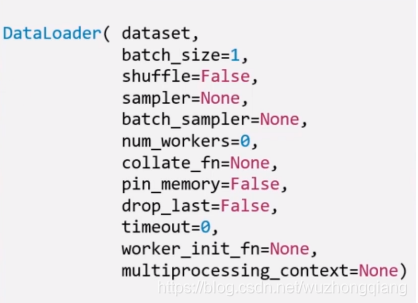• dataset: Dataset类， 决定数据从哪读取以及如何读取
• bathsize: 批大小
• num_works: 是否多进程读取机制
• shuffle: 每个epoch是否乱序
• drop_last: 当样本数不能被batchsize整除时， 是否舍弃最后一批数据

要理解这个drop_last， 首先，得先理解Epoch， Iteration和Batchsize的概念：

• Epoch： 所有训练样本都已输入到模型中，称为一个Epoch
• Iteration： 一批样本输入到模型中，称为一个Iteration
• Batchsize： 批大小， 决定一个Epoch有多少个Iteration

举个例子就Ok了， 假设样本总数80， Batchsize是8， 那么1Epoch=10 Iteration。 假设样本总数是87， Batchsize是8， 如果drop_last=True, 那么1Epoch=10Iteration, 如果等于False， 那么1Epoch=11Iteration, 最后1个Iteration有7个样本。
————————————————
原文链接：https://blog.csdn.net/wuzhongqiang/article/details/105499476

展开全文pytorch 人工智能 python

## Dataset

创建Dataset对象：
需要重写 getitem 方法和 len 方法。

from torch.utils.data import Dataset
# 输入形式：[{'x':['token_id',..],'y':[label]},..],[(['token_id',..],[label]),..]
class SampleDataset(Dataset):
def __init__(self,data_pair): #[([x],[y]),..]
self.x = [i for i in data_pair]
# [[x],..] shape:(len,seq)
self.y = [i for i in data_pair]
# [[y],..]
self.len = len(data_pair)
def __getitem__(self,index):
return self.x[index],self.y[index]
# 注意：返回时self.x和self.y长度要相同
def __len__(self):
return self.len


2.根据是否设置shuffle选择顺序采样器还是随机采样器
3.采样器根据数据集大小生成一个可迭代的序号列表[0,n-1]

# dataloader需接收tensor
dataset = SampleDataset(train_data) #获取可迭代对象


## collate_fn()

对batch排序

展开全文pytorch 深度学习 python

用于处理数据样本的代码可能会变得凌乱且难以维护；理想情况下，我们希望数据集代码与模型训练代码解耦，以获得更好的可读性和模块化。PyTorch提供的torch.utils.data.DataLoadertorch.utils.data.Dataset允许你使用预下载的数据集或自己制作的数据。Dataset用于存储样本及其相应的标签，而DataLoader能为数据集提供一个迭代器，以便于访问样本。

PyTorch域库提供了许多预加载的数据集（如FashionMNIST），且都是torch.utils.data.Dataset的子类。你可以在这里找到它们：图像数据集、文本数据集和音频数据集

## Dataset加载数据集

torchvision加载Fashion MNIST数据集为例。Fashion MNIST是Zalando文章里的图像数据集，包括60000个训练样本和10000个测试样本。每个示例包括一个28×28灰度图像(特征图)和10个类别之一的标签。

我们使用以下参数加载Fashion MNIST数据集：

• root是要存储训练/测试数据的路径

• train指定数据集为训练集或测试集，

• download=True表示如果在root无从获取数据集，则从网上下载。

• transformtarget_transform分别指定特征图和标签数据类型变换。

import torch
from torch.utils.data import Dataset
from torchvision import datasets
from torchvision.transforms import ToTensor,Lambda
import matplotlib.pyplot as plt
import numpy as np

training_data = datasets.FashionMNIST(
root="data",
train=True,
transform=ToTensor(),
target_transform = Lambda(lambda y: torch.zeros(
10, dtype=torch.float).scatter_(dim=0, index=torch.tensor(y), value=1))
)

test_data = datasets.FashionMNIST(
root="data",
train=False,
transform=ToTensor(),
target_transform = Lambda(lambda y: torch.zeros(
10, dtype=torch.float).scatter_(dim=0, index=torch.tensor(y), value=1))
)

运行以上程序， Out:

Downloading http://fashion-mnist.s3-website.eu-central-1.amazonaws.com/train-images-idx3-ubyte.gz
Extracting data/FashionMNIST/raw/train-images-idx3-ubyte.gz to data/FashionMNIST/raw

Extracting data/FashionMNIST/raw/train-labels-idx1-ubyte.gz to data/FashionMNIST/raw

Extracting data/FashionMNIST/raw/t10k-images-idx3-ubyte.gz to data/FashionMNIST/raw

Extracting data/FashionMNIST/raw/t10k-labels-idx1-ubyte.gz to data/FashionMNIST/raw

## transforms

数据被处理后的形式并不总是适合训练机器学习算法。所以，我们使用transforms对数据执行一些操作，使其适合于训练。

所有TorchVision数据集都有两个参数，其中transform用于修改特征图，target_transform用于修改标签。torchvision.transforms模块提供了几种常用的转换，如下文的ToTensor()Lambda

FashionMNIST的特征是PIL图像格式，标签是整数。对于训练，我们需要将特征规范化为张量（tensor），将标签用独热(one-hot)编码张量表示。为了进行这些转换，我们使用ToTensorLambda

### ToTensor()

ToSensor将PIL图像或NumPy ndarray转换为浮点张量(FloatTensor)。并图像的像素值在[限制在[0,1]范围内。

### Lambda Transforms

Lambda转换应用任何用户定义的lambda函数。在这里，我们定义了一个函数来将整数转换为一个独热编码张量。它首先创建一个大小为class_num的零张量（数据集中标签的数量），并调用scatter_，它在标签y给定的索引上指定值为1。

target_transform = Lambda(lambda y: torch.zeros(
class_num, dtype=torch.float).scatter_(dim=0, index=torch.tensor(y), value=1))

## 迭代和可视化数据集

我们可以像列表一样索引数据集：training_data[index]。我们使用matplotlib可视化训练数据中的一些样本。

labels_map = {
0: "T-Shirt",
1: "Trouser",
2: "Pullover",
3: "Dress",
4: "Coat",
5: "Sandal",
6: "Shirt",
7: "Sneaker",
8: "Bag",
9: "Ankle Boot",
}
figure = plt.figure(figsize=(8, 8))
cols, rows = 3, 3
for i in range(1, cols * rows + 1):
sample_idx = torch.randint(len(training_data), size=(1,)).item()
img, label = training_data[sample_idx]
print('one-hot：',label)
index=torch.nonzero(label)   # one-hot转整数标签
plt.title(labels_map[index.item()])
plt.axis("off")
plt.imshow(img.squeeze(), cmap="gray")
plt.show()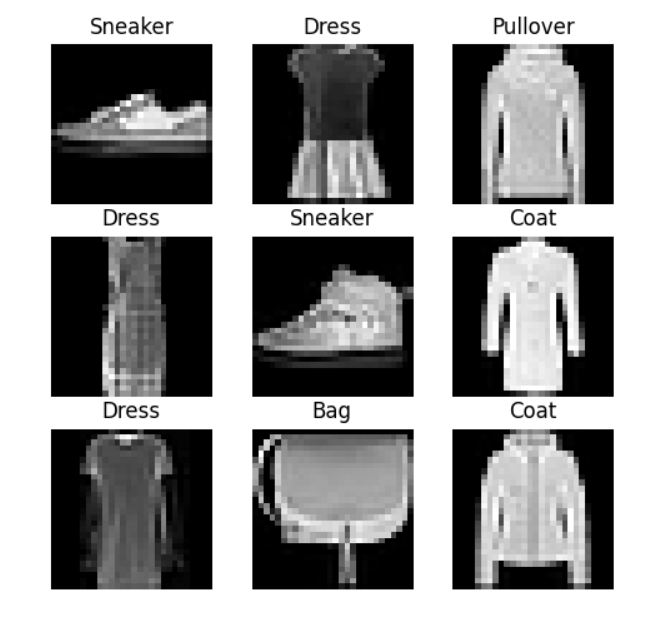one-hot：tensor([0., 0., 0., 0., 0., 0., 0., 1., 0., 0.])
one-hot：tensor([0., 0., 0., 1., 0., 0., 0., 0., 0., 0.])
one-hot：tensor([0., 0., 1., 0., 0., 0., 0., 0., 0., 0.])
one-hot：tensor([0., 0., 0., 1., 0., 0., 0., 0., 0., 0.])
one-hot：tensor([0., 0., 0., 0., 0., 0., 0., 1., 0., 0.])
one-hot：tensor([0., 0., 0., 0., 1., 0., 0., 0., 0., 0.])
one-hot：tensor([0., 0., 0., 1., 0., 0., 0., 0., 0., 0.])
one-hot：tensor([0., 0., 0., 0., 0., 0., 0., 0., 1., 0.])
one-hot：tensor([0., 0., 0., 0., 1., 0., 0., 0., 0., 0.])

## 自定义数据集类——真假索隆

首先，导入相关库：

import torch
from torchvision.transforms import ToTensor, ToPILImage, Lambda
import matplotlib.pyplot as plt
import os
import pandas as pd
from PIL import Image

图像所在文件夹与标签的Excel文件为：

img_path = '.\zoro'
label_path = '.\label_zoro.xlsx'

有10张图片，5张假(用0标记)5张真(用1标记)

链接：https://pan.baidu.com/s/1xGm6IMhq8zBQYZZCwwf3Aw 提取码：1111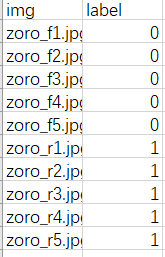自定义数据集类

自定义数据集类必须实现三个函数：__init__, __len__, 和__getitem__

class ZoroDataset(Dataset):
def __init__(self, label_file, img_dir, transform=None, target_transform=None):
# 读取标签文件
# 定义文件目录
self.img_dir = img_dir
# 定义transform
self.transform = transform
self.target_transform = target_transform

def __len__(self):
'''返回数据集中的样本数'''
return len(self.labels)

def __getitem__(self, index):
# 获取图片路径，0表示Excel文件的第一列
img_path = os.path.join(self.img_dir, self.labels.iloc[index, 0])
# 读取图片
image = Image.open(img_path)
# 获取图片对应的标签，1表示Excel文件的第二列
y_label = int(self.labels.iloc[index, 1])
# 如果使用时附加了transform参数，则对图片、标签应用转换
if self.transform:
image = self.transform(image)
if self.target_transform:
y_label = self.target_transform(y_label)
return image, y_label

定义一个图像展示函数

def img_show(img):
'''将img转化为PIL图像格式后展示'''
to_pil_image = ToPILImage()
img = to_pil_image(img)
plt.imshow(img)

定义一个索隆数据集实例

# 这里标签采用one-hot编码只是为了展示效果，不考虑实际意义
dataset = ZoroDataset(label_file=label_path, img_dir=img_path, transform=ToTensor(),
target_transform=Lambda(lambda y: torch.zeros(
2, dtype=torch.float).scatter_(dim=0, index=torch.tensor(y), value=1)))

读取图片和标签

train_features, train_labels = dataset
img_show(train_features)
print('one-hot标签：',train_labels)
plt.show()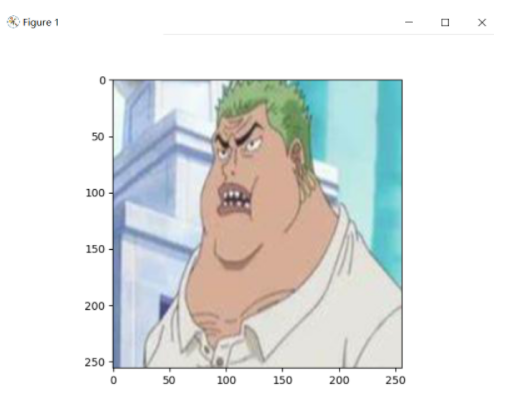输出：

one-hot标签：tensor([1., 0.])

Dataset一次检索只能检索一个样本。但我们在训练模型时，通常希望以“小批量”的方式采样，并在每个回合(epoch)打乱数据以避免模型过拟合。这就需要DataLoader了。DataLoader通过一个简单的API为我们抽象了这种复杂的功能，且是可迭代的。

batch_size = 2
print('数据集样本数：', len(dataset))  # __len__()

我们已经将该数据集加载到DataLoader中，接下来可以根据需要对数据集进行迭代。下面的例子中每次迭代都会返回一批train_featurestrain_labels（分别包含batch_size个特征和标签）。当我们指定了shuffle=True时，所以在对所有批进行迭代之后，数据顺序将被打乱。

可以用next()for循环对DataLoader进行迭代：

• next()

for epoch in range(2):
for i in range(int(len(dataset) / batch_size)):
train_features, train_labels = next(data_iter)
plt.figure(i)
for j in range(batch_size):
plt.subplot(int(f"1{batch_size}{j + 1}"))
# print(train_features.size())
img = train_features[j]
label = train_labels[j]
img_show(img)

print(f"Label: {label}")
plt.show()
• for循环

for epoch in range(2):
for i, (train_features, train_labels) in enumerate(data_iter):
plt.figure(i)
for j in range(batch_size):
plt.subplot(int(f"1{batch_size}{j + 1}"))
# print(train_features.size())
img = train_features[j]
label = train_labels[j]
img_show(img)

print(f"Label: {label}")
plt.show()

shuffle=True时，数据集被打乱：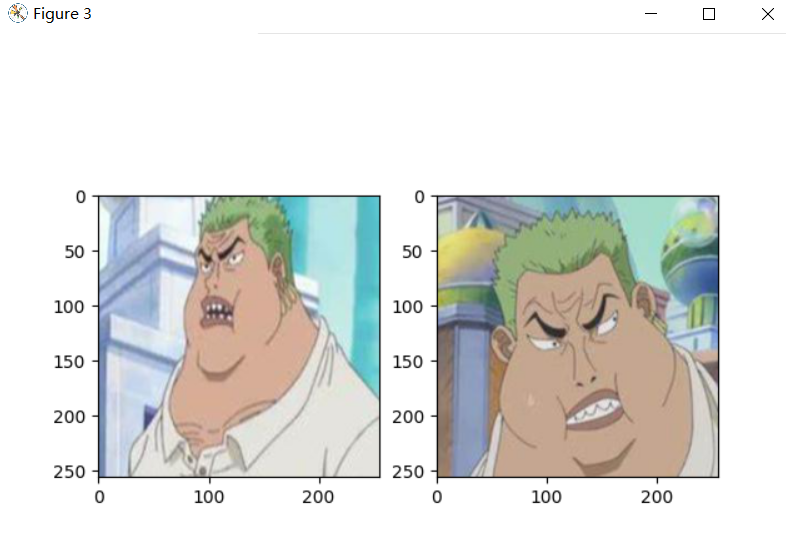shuffle=False时，按顺序迭代：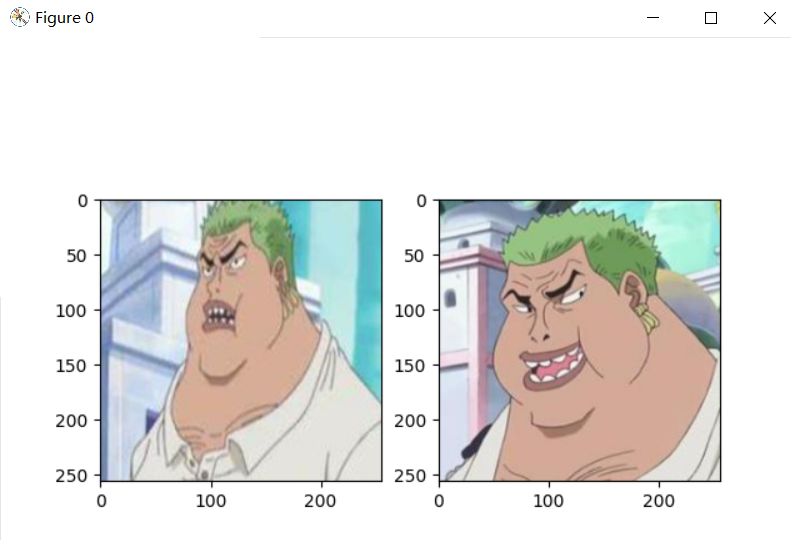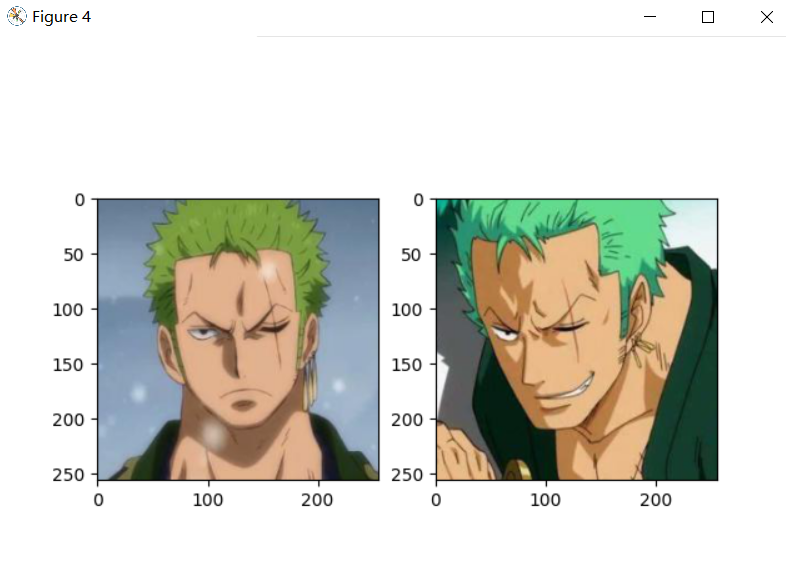全部代码：

import torch
from torchvision.transforms import ToTensor, ToPILImage, Lambda
import matplotlib.pyplot as plt
import os
import pandas as pd
from PIL import Image

img_path = '.\zoro'
label_path = '.\label_zoro.xlsx'

class ZoroDataset(Dataset):
def __init__(self, label_file, img_dir, transform=None, target_transform=None):
# 读取标签文件
# 定义文件目录
self.img_dir = img_dir
# 定义transform
self.transform = transform
self.target_transform = target_transform

def __len__(self):
'''返回数据集中的样本数'''
return len(self.labels)

def __getitem__(self, index):
# 获取图片路径，0表示Excel文件的第一列
img_path = os.path.join(self.img_dir, self.labels.iloc[index, 0])
# 读取图片
image = Image.open(img_path)
# 获取图片对应的标签，1表示Excel文件的第二列
y_label = int(self.labels.iloc[index, 1])
# 如果使用时附加了transform参数，则对图片应用转换
if self.transform:
image = self.transform(image)
if self.target_transform:
y_label = self.target_transform(y_label)
return image, y_label

def img_show(img):
'''将img转化为PIL图像格式后展示'''
to_pil_image = ToPILImage()
img = to_pil_image(img)
plt.imshow(img)

# 这里标签采用one-hot编码只是为了展示效果，不考虑实际意义
dataset = ZoroDataset(label_file=label_path, img_dir=img_path, transform=ToTensor(),
target_transform=Lambda(lambda y: torch.zeros(
2, dtype=torch.float).scatter_(dim=0, index=torch.tensor(y), value=1)))

train_features, train_labels = dataset
# img_show(train_features)
# print('one-hot标签：', train_labels)
# plt.show()
batch_size = 2
print('数据集样本数：', len(dataset))

for epoch in range(2):
for i, (train_features, train_labels) in enumerate(data_iter):
plt.figure(i)
for j in range(batch_size):
plt.subplot(int(f"1{batch_size}{j + 1}"))
# print(train_features.size())
img = train_features[j]
label = train_labels[j]
img_show(img)
print(f"Label: {label}")
plt.show()
展开全文算法 python 机器学习 深度学习 tensorflow
• 但是在深度学习中，数据量通常是都非常多，非常大的，如此大量的数据，不可能一次性的在模型中进行向前的计算反向传播，经常我们会对整个数据进行随机的打乱顺序，把数据处理成个个的batch,同时还会对数据进行...pytorch python 深度学习
• 目录前言DataSetDataLoader数据构建1. 创建Dataset 类 :sparkles:2. 读取数据 :ambulance:3. 返回数据 :zap:读取数据 :art:注意细节 :rocket:对比实验 前言 ???? 本章节主要介绍如何使用torch.utils.data 中的...pytorch 深度学习 人工智能
• 目录一、一级目录1.1 二级目录1.1.1 三级目录 一、一级目录 1.1 二级目录 1.1.1 三级目录pythonpytorch 深度学习 python
• Torchvison-dataset的使用 这里介绍的时是Torchvision中关于数据库Dataset的一些使用方法。 首先我们可以在Pytorch观望中看到Torchvision中的很多数据集： 以CIFAR为例，点进去后可以了解到更多关于该数据集的一些...pytorch 深度学习pytorch 深度学习 python 神经网络 人工智能
• Dataset和DataLoader都是Pytorch里面读取数据的工具。现在对这两种工具做一个概括总结。 1.Dataset 一个例子： # 导入需要的包 import torch import torch.utils.data.dataset as Dataset import numpy as np ...深度学习
• 在深度学习中，数据量通常是都非常多，非常大的，如此大量的数据，不可能一次性的在模型中进行向前的计算反向传播，经常我们会对整个数据进行随机的打乱顺序，把数据处理成一个个的batch，同时还会对数据进行...pytorch 深度学习 人工智能
• DataSet类的说明 An abstract class representing a :class:Dataset. All datasets that represent a map from keys to data samples should subclass it. All subclasses should overwrite :meth:__getitem__, ...pytorch 深度学习 python列表 数据结构 python 机器学习 人工智能pycharm 深度学习 python
• 文章目录：1 Dataset基类2 构建Dataset子类2.1 __Init__2.2 __getitem__3 dataloader 1 Dataset基类 PyTorch 读取其他...人工智能 深度学习 python tensorflow 机器学习
• 2.2 utils.data.DataLoader2.3 下面介绍一下自定义数据集构成方法类型1：map-style datasets类型2：iterable-style datasetsReference: 1.数据处理工具箱概述 数据下载预处理是机器学习、深度学习实际项目中...python pytorch 神经网络 深度学习 机器学习pytorch
• 在学习深度学习初期，被各种算法、各种名词吸引，学习了一些相关知识、框架方法，也跑过Mnist分类以及iris回归。但是当真正拿到一个陌生的数据集时，需要重头开始搭建一个完整的模型时，常常会感觉到无从下手，...pytorchpytorch 深度学习 python
• class Dataset(Generic[T_co]): r"""一个抽象类表示为:class: ' Dataset '。 所有表示从键到数据样本映射的数据集都应该子类化它。 所有子类都应该覆写:方法: ' __getitem__ '， 支持获取已给定键的数据样本。...python人工智能 python 深度学习
• 实现方法 导入 from torch.utils.data import Dataset, DataLoader Dataset torch内置抽象类，无法实例化，通过继承并重写魔术方法实现 class MyDataset(Dataset): def __init__(self, filepath): xy = np.load...pytorch 深度学习 python算法 机器学习 深度学习 人工智能...